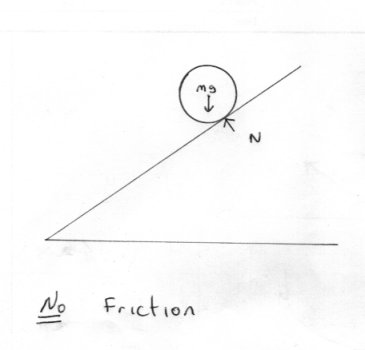Rolling

Recommended Posts

Hello,

I'm new here.

Can anyone check these equations if they are correct.

Rolling velocity

Without friction - v=gt sin x

With friction - v=gt(sin x- ucos x)

x is angle of inclination and u is frictional coefficient and t is time taken and g is acceleration due to gravity.

Share on other sites

Is this homework?

You really need to explain the problem more completely otherwise people are left guessing what you mean.

I am guessing that you are talking about a ball rolling down a slope from a standing start so the equation of constant acceleration applies with the downslope acceleration = gsin(x) so we have using

vt = u + ft = 0 + gsin(x) * t

But will the ball roll or slide down the slope?

Share on other sites

Hello, studiot, the ball is rolling down the slope. They aren't any homework, I did try to find rolling equations myself and put them here for check.

Share on other sites

You gave two situations I started with the first and simplest.

What causes the ball to roll if there is no friction?In the real world, air friction would be enough to turn it, but in our idealisation there are no such forces acting.

Note that a vehicle or railway engine wheels will spin under the vehicle when driven from the centre axle without moving the vehicle if there is not enough friction.

Share on other sites

Is this homework?

You really need to explain the problem more completely otherwise people are left guessing what you mean.

I am guessing that you are talking about a ball rolling down a slope from a standing start so the equation of constant acceleration applies with the downslope acceleration = gsin(x) so we have using

vt = u + ft = 0 + gsin(x) * t

But will the ball roll or slide down the slope?

" vt = u + ft = 0 + gsin(x) * t "

Surely that would be the equation of a block/ball sliding down a frictionless slope. A ball rolling down a slope has a complete other factor to be considered - which your other points show you are keeping in mind - and which I will not spoil the fun by mentioning.

To give a hint: create your model based on conservation of energy, consider at start of experiment a still ball at height h1 and at the end a rolling ball at h2.

Hint 2 - it is not the same as the frictionless slope equation given above

Share on other sites

Surely that would be the equation of a block/ball sliding down a frictionless slope.

Yes that is what I said.

I also asked what causes the ball to roll if there is no friction?

Share on other sites

Well, friends, I think I should show the derivation of these equations here.

Check this: https://en.wikipedia.org/wiki/Rolling

Check whether the last section of the article (created by me) is right.

Share on other sites

You're editing wikipedia without the first idea of the topic?

Think of a frictionless slope and a natural frictional slope. In one case the ball will slide down - in the second it will roll down. They are NOT the same situation. I suggested an energy audit - did you give it a go? Did you answer Studiot's question of why the ball rolls?

Share on other sites

Certainly, the body will slide if there is no friction. But, we think that some imaginary force F acts on the body thereby creating a couple, causing rotation, we can surely accept it as rolling without friction. I agree that situation is only imaginable and not practical. For the real world, the second equation should be apt.

Share on other sites

Certainly, the body will slide if there is no friction. But, we think that some imaginary force F acts on the body thereby creating a couple, causing rotation, we can surely accept it as rolling without friction. I agree that situation is only imaginable and not practical. For the real world, the second equation should be apt.

Nah. A body that goes from stationary to rotating (whether or not there is additional linear motion as well) has changed measurably - imaginary forces do not cut it; you need to have force applied over a distance and thus work to do an energy audit. If it is sliding with no friction, or sliding with some friction then you have one simple case - but rolling brings a completely new element to the model which must be accounted for properly; you have not done this

Share on other sites

Great Find. I always like explanations that use two different ways to get to the same answer. Out of curiosity - I would use the second method; am I right in presuming you would have used the first?

Share on other sites

I seeeeeeeeeeeeeeeeeeeeeee....................So, are my equations wrong ?Create an account

Register a new account